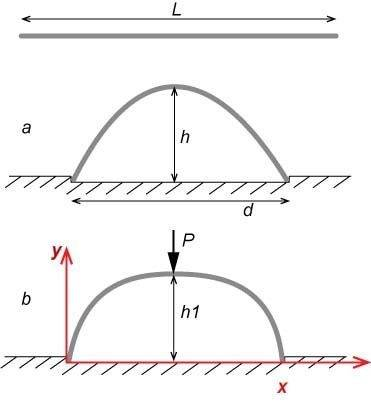# Liner buckling## Homework Statement

A beam (e.g. a steel liner) of a length L is bent between two supports, as shown in fig (a).
According to the Euler column, the shape is a half period of a sine.
Now, a force P is applied at the axis of symmetry - fig (b).
What is the shape of the liner? y=f(x)
Instead of the general case, let's choose P so weak that the liner remains always convex (h1=0.9*h or so ...).

## The Attempt at a Solution

I followed the "Strength of Materials and Structures" of Case-Chilver-Ross (ISBN:0340719206)
and tried to modify the 18.9 - "Strut with uniformly distributed lateral loading"
to a "... with concentrated lateral load" .
How to apply the boundary conditions so that the length of the liner (arc length) L remains constant?

OldEngr63
Gold Member
What value is the ratio h/d for your problem? If the ratio is very large, your sinusoidal shape is not correct.

Agreed. But this way the drawing is more clear.
Is there a solution when h/d is:
a) so low that sine shape stands?
b) so large that it is not a sine any more?